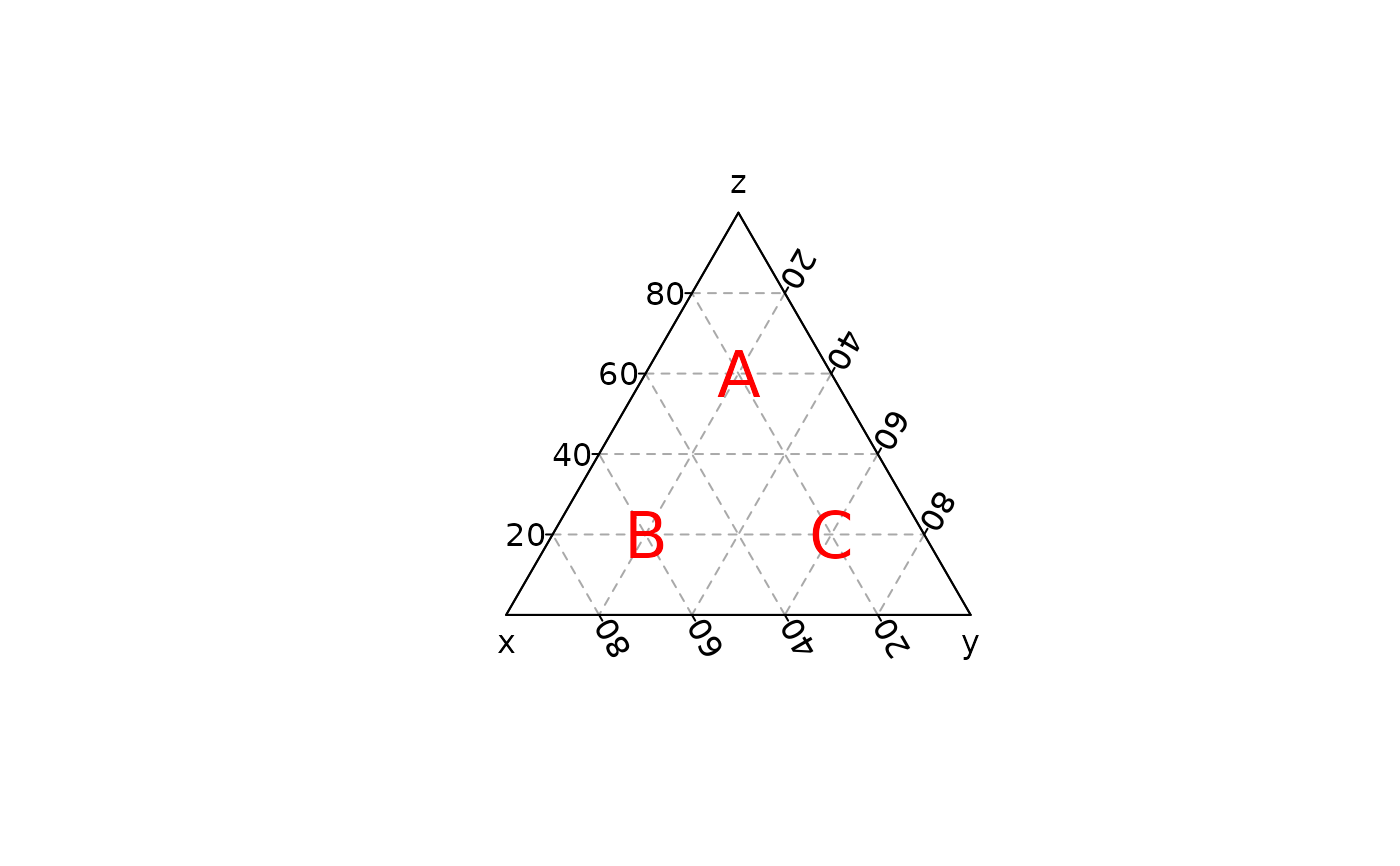Draws the strings given in the vector labels at the coordinates given by x, y and z.

## Usage

ternary_text(x, y, z, ...)

# S4 method for numeric,numeric,numeric
ternary_text(x, y, z, labels = seq_along(x), ...)

# S4 method for ANY,missing,missing
ternary_text(x, labels = seq_along(x\$x), ...)

## Arguments

x, y, z

A numeric vector giving the x, y and z ternary coordinates of a set of points. If y and z are missing, an attempt is made to interpret x in a suitable way (see grDevices::xyz.coords()).

...

Further arguments to be passed to graphics::text().

labels

A character vector or expression specifying the text to be written.

## Value

ternary_text() is called it for its side-effects.

graphics::text()

Other geometries: ternary_arrows(), ternary_crosshairs(), ternary_lines(), ternary_points(), ternary_polygon(), ternary_segments()

N. Frerebeau

## Examples

## Compositional data
coda <- data.frame(
X = c(20, 60, 20),
Y = c(20, 20, 60),
Z = c(60, 20, 20)
)